International
Tables for
Crystallography
Volume B
Reciprocal space
Edited by U. Shmueli

International Tables for Crystallography (2010). Vol. B, ch. 1.5, p. 190   | 1 | 2 |

## Section 1.5.5.4.2. Splitting of k-vector types

M. I. Aroyoa* and H. Wondratschekb

aDepartamento de Fisíca de la Materia Condensada, Facultad de Cienca y Technología, Universidad del País Vasco, Apartado 644, 48080 Bilbao, Spain , and bInstitut für Kristallographie, Universität, D-76128 Karlsruhe, Germany
Correspondence e-mail:  wmpararm@lg.ehu.es

#### 1.5.5.4.2. Splitting of k-vector types

| top | pdf |

The Brillouin zone as well as the unit cell are always convex bodies; the same holds for the representation domain of CDML and for the choice of the asymmetric unit. It is thus sometimes unavoidable that the k-vector types are split and that the different parts belong to different arms and to different stars of k vectors. Sometimes this splitting of k-vector types may be avoided by an appropriate choice of the asymmetric unit; sometimes the introduction of flagpoles and wings is necessary to make the k-vector types uni-arm.

#### Examples

 (1) In the reciprocal-space group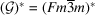, No. 225 of the arithmetic crystal class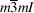there are the lines of k vectors Λ (α, α, α) and F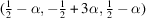of CDML, p. 41. By Figure 1.5.5.1one sees that the line Λ connects the points Γ and P, the line F connects the points P and H. One takes from the corresponding Table 1.5.5.1the coefficients of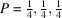and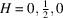. From these points or from the transformation listed at the top of Table 1.5.5.1as `Parameter relations' the coefficients of the line F are obtained as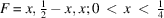. The inspection of the symmetry diagram of, No. 225, in IT A shows that a twofold rotation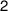(represented by the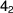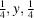screw-rotation axis) leaves the point P invariant, whereas the point H is mapped onto the point R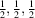. More formally: the rotation is described by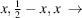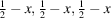, where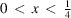. The result is the line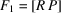. It is uni-arm to the line Λ = x, x, x and the union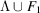forms the Wintgen position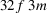. An analoguous result is obtained for the same lines in the arithmetic crystal class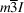. (2) In the following example the splitting of a Wintgen position happens if a representation domain of the Brillouin zone is chosen. The splitting can be avoided by the choice of the asymmetric unit. We consider the planein the arithmetic crystal class, see Fig. 1.5.5.4and Table 1.5.5.4. In CDML this plane is split into the parts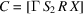and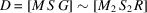. By the choice of the asymmetric unit the independent region of the Wintgen position is uni-arm: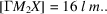: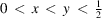. (3) The splitting of a Wintgen position can be avoided if flagpoles and wings are admitted, i.e. if the minimal domain is described by a non-convex body. If one chooses in the first example of the arithmetic crystal classesandthe unionfor the line, then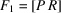forms a flagpole, whereas Λ forms an edge of the asymmetric unit, see Figs. 1.5.5.1and 1.5.5.2. The same holds for the Wintgen position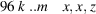of. In the representation domain which is simultaneously the asymmetric unit, this Wintgen position is split into three parts B, C and J, which form three of the four walls of the (tetrahedral) minimal domain. By proper symmetry operations these three parts can be made uni-arm to the part C, such that their union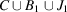describes the independent part of that Wintgen position, see Fig. 1.5.5.1. The part C forms a wall of the asymmetric unit; the part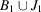forms a wing, see Fig. 1.5.5.1.